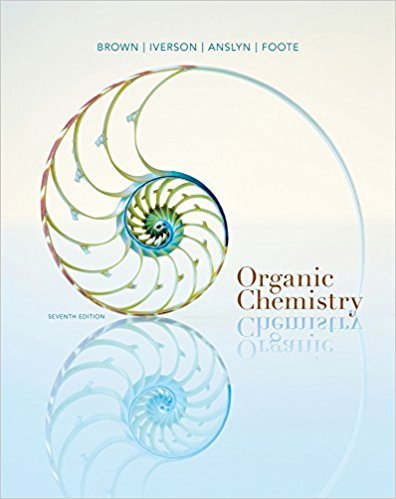×
Get Full Access to Organic Chemistry - 7 Edition - Chapter 10 - Problem 10.27
Get Full Access to Organic Chemistry - 7 Edition - Chapter 10 - Problem 10.27

×

# From each pair, select the stronger base. For each stronger base, write a structuralISBN: 9781133952848 483

## Solution for problem 10.27 Chapter 10

Organic Chemistry | 7th Edition

• Textbook Solutions
• 2901 Step-by-step solutions solved by professors and subject experts
• Get 24/7 help from StudySoup virtual teaching assistantsOrganic Chemistry | 7th Edition

4 5 1 326 Reviews
27
4
Problem 10.27

From each pair, select the stronger base. For each stronger base, write a structural for-mula of its conjugate acid.(a) OH2 or CH3O2 (each in H2O) (b) CH3CH2O2 or CH3C#C2(c) CH3CH2S2 or CH3CH2O2 (d) CH3CH2O2 or NH2

Step-by-Step Solution:
Step 1 of 3

Wednesday, February 8th Chapter 4 - Chemical quantities and Aqueous Reactions Types of reactions : Gas Evolution, Acid, and Combustion Oxygen and Glucose are Reactants of combustion, while water and Carbon dioxide are Products. Remember the Law of Conservation of Mass - this is why we have to balance equations. When balancing equations, balance the compounds in which the elements that appear the least ﬁrst. Limiting Reactants - If not stated, assume that there are inﬁnite amount of all [other] reactants present. 1. Balance equation 2. Convert grams to moles (moles is equal to the mass divided by the molecular weight) 3. Do mole to mole conversations 4. Determine the limiting reagent (smallest amount of product formed) 5. Convert back to grams Theoretical and actual yield limiting reagent - the reagent that determines the maximum amount of theoretical yield Theoretical yield - expected yield from quantities of reactants Actual yield: The quantity of product actually produced Percent yield - actual/theoretical x 100 do exercise Friday, February 10th Molecular weights don’t factor into equations in terms of signiﬁcant ﬁgures Limiting reactant exercises** Percent yield exercises* Molarity (M) or concentration (c) = Moles of solute (n)/ Volume of solution in LITERS (v) Solute - What is being dissolved Solvent - What the solute is being dissolved in Solution - Homogeneous mixture. Every solution has a solute and a solvent Solution preparation - with two liquids, the solvent is whichever liquid is in excess; if 50%-50%, whichever liq

Step 2 of 3

Step 3 of 3

##### ISBN: 9781133952848

This textbook survival guide was created for the textbook: Organic Chemistry, edition: 7. The answer to “From each pair, select the stronger base. For each stronger base, write a structural for-mula of its conjugate acid.(a) OH2 or CH3O2 (each in H2O) (b) CH3CH2O2 or CH3C#C2(c) CH3CH2S2 or CH3CH2O2 (d) CH3CH2O2 or NH2” is broken down into a number of easy to follow steps, and 36 words. Since the solution to 10.27 from 10 chapter was answered, more than 357 students have viewed the full step-by-step answer. The full step-by-step solution to problem: 10.27 from chapter: 10 was answered by , our top Chemistry solution expert on 03/16/18, 05:02PM. This full solution covers the following key subjects: . This expansive textbook survival guide covers 24 chapters, and 1131 solutions. Organic Chemistry was written by and is associated to the ISBN: 9781133952848.

## Discover and learn what students are asking

Statistics: Informed Decisions Using Data : Scatter Diagrams and Correlation
?A _______ variable is a variable that is related to both the explanatory and response variable.

Statistics: Informed Decisions Using Data : Inference about the Difference between Two Medians: Independent Samples
?In Problems 1–8, use the Mann–Whitney test to test the given hypotheses at the = 0.05 level of significance. The independent samples were obtained ra

Unlock Textbook Solution